Dave's Math Tables: Series Convergence Tests(Math | Calculus | Expansions | Series | Convergence Tests)

Definition of Convergence and Divergence in Series
The nth partial sum of the series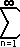an is given by Sn = a1 + a2 + a3 + ... + an. If the sequence of these partial sums {Sn} converges to L, then the sum of the series converges to L. If {Sn} diverges, then the sum of the series diverges.

Operations on Convergent Series

If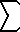an = A, andbn = B, then the following also converge as indicated:can = cA(an + bn) = A + B(an - bn) = A - B

Alphabetical Listing of Convergence Tests

Absolute Convergence

If the series|an| converges, then the seriesan also converges.
Alternating Series Test
If for all n, an is positive, non-increasing (i.e. 0 < an+1 <= an), and approaching zero, then the alternating series(-1)n an   and(-1)n-1 an
both converge.
If the alternating series converges, then the remainder RN = S - SN (where S is the exact sum of the infinite series and SN is the sum of the first N terms of the series) is bounded by |RN| <= aN+1

Deleting the first N Terms
If N is a positive integer, then the seriesan and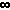an   n=N+1
both converge or both diverge.

Direct Comparison Test
If 0 <= an <= bn for all n greater than some positive integer N, then the following rules apply:
Ifbn converges, thenan converges.
Ifan diverges, thenbn diverges.

Geometric Series Convergence

The geometric series is given by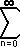a rn = a + a r + a r2 + a r3 + ...
If |r| < 1 then the following geometric series converges to a / (1 - r).

If |r| >= 1 then the above geometric series diverges.

Integral Test
If for all n >= 1, f(n) = an, and f is positive, continuous, and decreasing thenan and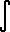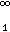an
either both converge or both diverge.
If the above series converges, then the remainder RN = S - SN (where S is the exact sum of the infinite series and SN is the sum of the first N terms of the series) is bounded by 0< = RN <=(N..) f(x) dx.
Limit Comparison Test
If lim (n-->) (an / bn) = L,
where an, bn > 0 and L is finite and positive,
then the seriesan andbn either both converge or both diverge.

nth-Term Test for Divergence

If the sequence {an} does not converge to zero, then the seriesan diverges.

p-Series Convergence
The p-series is given by1/np = 1/1p + 1/2p + 1/3p + ...
where p > 0 by definition.
If p > 1, then the series converges.
If 0 < p <= 1 then the series diverges.
Ratio Test
If for all n, n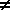0, then the following rules apply:
Let L = lim (n -- >) | an+1 / an |.
If L < 1, then the seriesan converges.
If L > 1, then the seriesan diverges.
If L = 1, then the test in inconclusive.
Root Test
Let L = lim (n -- >) | an |1/n.
If L < 1, then the seriesan converges.
If L > 1, then the seriesan diverges.
If L = 1, then the test in inconclusive.
Taylor Series Convergence
If f has derivatives of all orders in an interval I centered at c, then the Taylor series converges as indicated:(1/n!) f(n)(c) (x - c)n = f(x)
if and only if lim (n-->) Rn = 0 for all x in I.
The remainder RN = S - SN of the Taylor series (where S is the exact sum of the infinite series and SN is the sum of the first N terms of the series) is equal to (1/(n+1)!) f(n+1)(z) (x - c)n+1, where z is some constant between x and c.